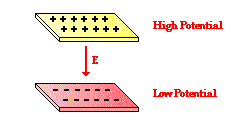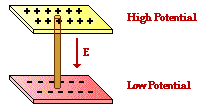Electric Circuits - Lesson 2 - Electric Current

# What is an Electric Circuit?

In Lesson 1, the concept of electric potential difference was discussed. Electric potential is the amount of electric potential energy per unit of charge that would be possessed by a charged object if placed within an electric field at a given location. The concept of potential is a location-dependent quantity - it expresses the quantity of potential energy on a per charge basis such that it is independent on the amount of charge actually present on the object possessing the electric potential. Electric potential difference is simply the difference in electric potential between two different locations within an electric field.To illustrate the concept of electric potential difference and the nature of an electric circuit, consider the following situation. Suppose that there are two metal plates oriented parallel to each other and each being charged with an opposite type of charge - one being positive and the other being negative. This arrangement of charged plates would create an electric field in the region between the plates that is directed away from the positive plate and towards the negative plate. A positive test charge placed between the plates would move away from the positive plate and towards the negative plate. This movement of a positive test charge from the positive plate to the negative plate would occur without the need of energy input in the form of work; it would occur naturally and thus lower the potential energy of the charge. The positive plate would be the high potential location and the negative plate would be the low potential location. There would be a difference in electric potential between the two locations.

Now suppose that the two oppositely charged plates are connected by a metal wire. What would happen? The wire serves as a sort of charge pipe through which charge can flow. Over the course of time, one could think of positive charges moving from the positive plate through the charge pipe (wire)to the negative plate. That is, positive charge would naturally move in the direction of the electric field that had been created by the arrangement of the two oppositely charged plates. As a positive charge leaves the upper plate, the plate would become less positively charged as illustrated in the animation at the right. As a positive charge reaches the negative plate, that plate would become less negatively charged. Over the course of time, the amount of positive and negative charge on the two plates would slowly diminish. Since the electric field depends upon the amount of charge present on the object creating the electric field, the electric field created by the two plates would gradually diminish in strength over the course of time. Eventually, the electric field between the plates would become so small that there would be no observable movement of charge between the two plates. The plates would ultimately lose their charge and reach the same electric potential. In the absence of an electric potential difference, there will be no charge flow.

The above illustration comes close to demonstrating the meaning of an electric circuit. However, to be a true circuit, charges must continually flow through a complete loop, returning to their original position and cycling through again. If there were a means of moving positive charge from the negative plate back up onto the positive plate, then the movement of positive charge downward through the charge pipe (i.e., the wire) would occur continuously. In such a case, a circuit or loop would be established.

A common lab activity that illustrates the necessity of a complete loop utilizes a battery pack (a collection of D cells), a light bulb, and some connecting wires. The activity involves observing the effect of connecting and disconnecting a wire in a simple arrangement of the battery pack, light bulbs and wires. When all connections are made to the battery pack, the light bulb lights. In fact, the lighting of the bulb occurs immediately after the final connection is made. There is no perceivable time delay between when the last connection is made and when the light bulb is perceived to light up.The fact that the light bulb lights and remains lit is evidence that charge is flowing through the light bulb filament and that an electric circuit has been established. A circuit is simply a closed loop through which charges can continuously move. To demonstrate that charges are not only moving through the light bulb filament but also through the wires connecting the battery pack and the light bulb, a variation on the above activity is made. A compass is placed beneath the wire at any location such that its needle is placed in alignment with the wire. Once the final connection is made to the battery pack, the light bulb lights and the compass needle deflects. The needle serves as a detector of moving charges within the wire. When it deflects, charges are moving through the wire. And if the wire is disconnected at the battery pack, the light bulb is no longer lit and the compass needle returns to its original orientation. When the light bulb lights, charge is moving through the electrochemical cells of the battery, the wires and the light bulb filaments; the compass needle detects the movement of this charge. It can be said that there is a current - a flow of charge within the circuit.The electric circuit demonstrated by the combination of battery, light bulb and wires consists of two distinct parts: the internal circuit and the external circuit. The part of the circuit containing electrochemical cells of the battery is the internal circuit. The part of the circuit where charge is moving outside the battery pack through the wires and the light bulb is the external circuit. In Lesson 2, we will focus on the movement of charge through the external circuit. In the next part of Lesson 2 we will explore the requirements that must be met in order to have charge flowing through the external circuit.

Next Section: